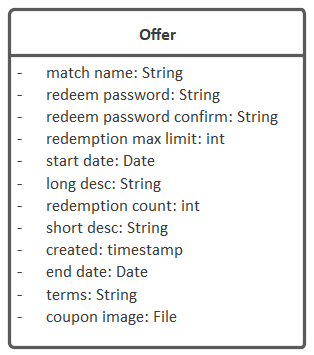# Reference - Attribute Data Types

Here’s a typical domain class added in Enterprise Architect (EA):The attribute types (`string`, `int` etc) do have an influence on the generated system, so it’s important to give some consideration to the data types when adding attributes to your domain class.

# Data types recognised by CodeBot

The following data types are recognised by CodeBot. As with “everything CodeBot”, this list grows over time; however we’ll keep this page updated with all the supported data types:

Data type Synonyms Notes
`boolean` `bool`
`string` `char`, `char[]`
`integer` `int`
`long`
`float`
`double` `number`, `decimal` Depending on the output target (e.g. JavaScript), all the numeric types may resolve to a common type such as “number”
`date`   Just the date with no time
`time`   Time of day with no date
`datetime` `timestamp` Date and time
`file`   Triggers a file upload capability in the REST API. The value stored in the record is simply the original filename.

All the data types are case insensitive - so you can define them in your model as `Bool`, `BOOL`, `bool` etc.

# Types mapped to output targets

Each data type is mapped to an equivalent type in the output target (language, database etc).

## MongoDB (BSON) types

Data type BSON type
`boolean` `bool`
`string` `string`
`integer` `int`
`long` `long`
`float` `decimal`
`double` `decimal`
`date` `date`
`datetime` `date`
`time` `date`

## SQL types

Data type SQL type
`boolean` `BOOLEAN`
`text` `TEXT`
`string` `VARCHAR(255)`
`integer` `INT`
`long` `BIGINT`
`float` `FLOAT`
`double` `DOUBLE`
`date` `DATE`
`datetime` `DATETIME`
`time` `TIME`
`timestamp` `TIMESTAMP`

## JSON types

Data type JSON type
`boolean` `boolean`
`string` `string`
`integer` `integer`
`long` `long`
`float` `number`
`double` `number`
`date` `string`
`datetime` `string`
`time` `string`

## Java types

Data type Java type
`boolean` `boolean`
`string` `String`
`integer` `int`
`long` `long`
`float` `BigDecimal`
`double` `BigDecimal`
`date` `java.time.ZonedDateTime`
`datetime` `string`
`time` `string`